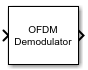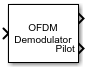# OFDM Demodulator Baseband

Demodulate using OFDM method

•Libraries:
Communications Toolbox / Modulation / Digital Baseband Modulation / OFDM

## Description

The OFDM Demodulator Baseband block demodulates an input time domain signal by using the orthogonal frequency division multiplexing (OFDM) method. For more information, see OFDM Demodulation. The block accepts a single input and has one or two output ports, depending on the status of Pilot output port.

This icon shows the block with all ports enabled.## Examples

expand all

The model filters an oversampled OFDM modulated signal through a single-input single-output (SISO) channel. After channel filtering, it demodulates the signal and compares the original data to the demodulated output.The `cm_oversample_ofdm_siso` model:

• Generates random integer data and pilot input symbols.

• 16-QAM modulates the data and pilot symbols.

• OFDM-modulates the QAM-modulated signal. The OFDM modulator and demodulator pair process three symbols, with different pilot subcarrier indices and cyclic prefix lengths for each symbol. The OFDM signal contains data and pilots that the model generates at four times the sample rate.

• Filters the OFDM-modulated signal through a SISO AWGN channel.

• OFDM-demodulates and separately outputs the data and pilot signals.

• 16-QAM demodulates to the data and pilot symbols.

• Computes symbol error rates for the data and pilot signals by using Error Rate Calculation blocks.

The model initializes variables used to configure block parameters by using the `PreLoadFcn` callback function. For more information, see Model Callbacks (Simulink).

Display the data and pilot symbol error rates.

```The data had a 0.014533 symbol error rate for 126126 samples. The pilots had a 0.014902 symbol error rate for 12012 samples. ```

The RMS block measures the OFDM-modulated signal scaled by a value proportional to the number of active subcarriers relative to the FFT size to confirm the signal power is approximately unity.

```The measured RMS value is 0.98499. ```

## Ports

### Input

expand all

OFDM-modulated baseband signal, specified as a (NCPTotal + NFFT × NSym)-by-NR matrix.

Data Types: `double`

### Output

expand all

Output baseband signal, returned as a matrix or NData-by-NSym-by-NR array. The output reduces to a matrix when NR is `1`.

• NData is the number of data subcarriers, such that NData = NFFTNleftGNrightGNDCNullNPilotNCustNull.

• NFFT is the number of subcarriers, as specified by FFT length.

• NleftG is the number of subcarriers in the left guard band, as specified by the first element of Number of guard bands.

• NrightG is the number of subcarriers in the right guard band, as specified by the second element of Number of guard bands.

• NDCNull is the number of subcarriers in the DC null, specified as `0` or `1` by selection of Remove DC carrier.

• NPilot is the number of pilot subcarriers in each symbol.

• NCustNull is the number of subcarriers used for custom nulls. To use custom nulls, you must specify Pilot subcarrier indices as a 3D array.

• NSym is the number of symbols, as specified by Number of OFDM symbols.

• NR is the number of receive antennas, as specified by Number of receive antennas.

Data Types: `double`
Complex Number Support: Yes

Pilot signal, returned as an NPilot-by-NSym-by-NR array.

#### Dependencies

To return this output, select Pilot output port.

Data Types: `double`
Complex Number Support: Yes

## Parameters

expand all

To edit block parameters interactively, use the Property Inspector. From the Simulink® Toolstrip, on the Simulation tab, in the Prepare gallery, select .

Number of FFT points, specified as a positive, integer scalar. The length of the FFT must be greater than or equal to 8 and is equivalent to the number of subcarriers.

Number of subcarriers allocated to the left and right guard bands, specified as a 2-by-1 integer vector. The number of left and right guard-band subcarriers, [NleftG; NrightG], must fall within [0,NFFT/2⌋ − 1], where NFFT is the total number of subcarrier in the OFDM signal specified by FFT length. For more information, see Subcarrier Allocation, Guard Bands, and Guard Intervals.

Select this parameter to remove the null DC subcarrier. The null DC subcarrier is located at the center of the frequency band and has the index value:

• (NFFT / 2) + 1 when NFFT is even.

• (NFFT + 1) / 2 when NFFT is odd.

NFFT is the total number of subcarriers in the OFDM signal specified by FFT length.

Select this parameter to add a port to output pilot subcarriers. When you set this parameter to:

• `off` — Pilot information may be present but remains embedded in the output data.

• `on` — The block separates the subcarriers, specified by Pilot subcarrier indices, from the output data and outputs the demodulated pilot signal to the Pilot port.

Indices of the pilot subcarrier locations, specified as a column vector, matrix, or 3D array with integer-element values in the range

`$\left[{N}_{\text{leftG}}+1,\text{\hspace{0.17em}}{N}_{\text{FFT}}/2\right]\cup \left[{N}_{\text{FFT}}/2+2,\text{\hspace{0.17em}}{N}_{\text{FFT}}-{N}_{\text{rightG}}\right],$`

where NFFT is the total number of subcarriers specified by FFT length, and NleftG and NrightG are the left and right guard bands specified by Number of guard bands.

You can assign the NPilot pilot carrier indices to the same or different NSym subcarriers for each symbol, and across NT transmit antennas.

• When the pilot indices are the same for every symbol and transmit antenna, the parameter has dimensions of NPilot-by-1.

• When the pilot indices vary across symbols, the parameter has dimensions of NPilot-by-NSym.

• If the received signal assigned one symbol across multiple transmit antennas, the parameter has dimensions of NPilot-by-1-by-NT.

• If the indices vary across the number of symbols and transmit antennas, the parameter has dimensions of NPilot-by-NSym-by-NT.

Tip

To minimize interference between transmissions across more than one transmit antenna, the pilot indices per symbol must be mutually distinct across the antennas.

#### Dependencies

This parameter applies when you select Pilot output port.

Length of the cyclic prefix for each OFDM symbol, specified as a positive, integer scalar or row vector containing Number of OFDM symbols elements. When you specify the cyclic prefix length as a:

• Scalar — The cyclic prefix length is the same for all symbols through all antennas.

• Row vector — The cyclic prefix length may vary across symbols but does not vary across antennas.

Oversampling factor, specified as a positive scalar. The oversampling factor must satisfy these constraints:

Tip

If you set the oversampling factor to an irrational number, specify the fractional value. For example, with an FFT length of `12` and an oversampling factor of `4/3`, their product is the integer `16`. However, rounding `4/3` to `1.333` when setting the oversampling factor results in a noninteger product of `15.9960`, which results in a code error.

Number of OFDM symbols in the time-frequency grid, specified as a positive, integer scalar.

Number of receive antennas to receive the OFDM modulated signal, specified as a positive, integer scalar less than or equal to `64`.

Type of simulation to run, specified as ```Interpreted execution``` or `Code generation`.

• `Interpreted execution` — Simulate the model by using the MATLAB® interpreter. This option requires less startup time, but the speed of subsequent simulations is slower than with the ```Code generation``` option. In this mode, you can debug the source code of the block.

• `Code generation` — Simulate the model by using generated C code. The first time you run a simulation, Simulink generates C code for the block. The model reuses the C code for subsequent simulations unless the model changes. This option requires additional startup time, but the speed of the subsequent simulations is faster than with the `Interpreted execution` option.

## Block Characteristics

 Data Types `double` Multidimensional Signals `yes` Variable-Size Signals `no`

## Algorithms

expand all

 Dahlman, E., S. Parkvall, and J. Skold. 4G LTE/LTE-Advanced for Mobile Broadband.London: Elsevier Ltd., 2011.

 Andrews, J. G., A. Ghosh, and R. Muhamed, Fundamentals of WiMAX, Upper Saddle River, NJ: Prentice Hall, 2007.

 IEEE Standard 802.16-2017. "Part 16: Air Interface for Broadband Wireless Access Systems." March 2018.